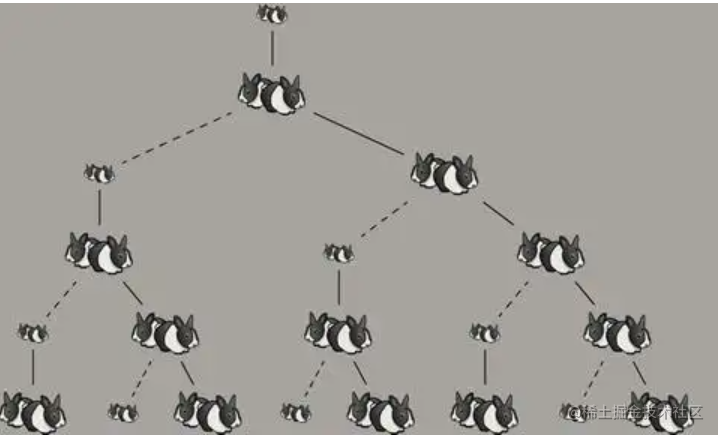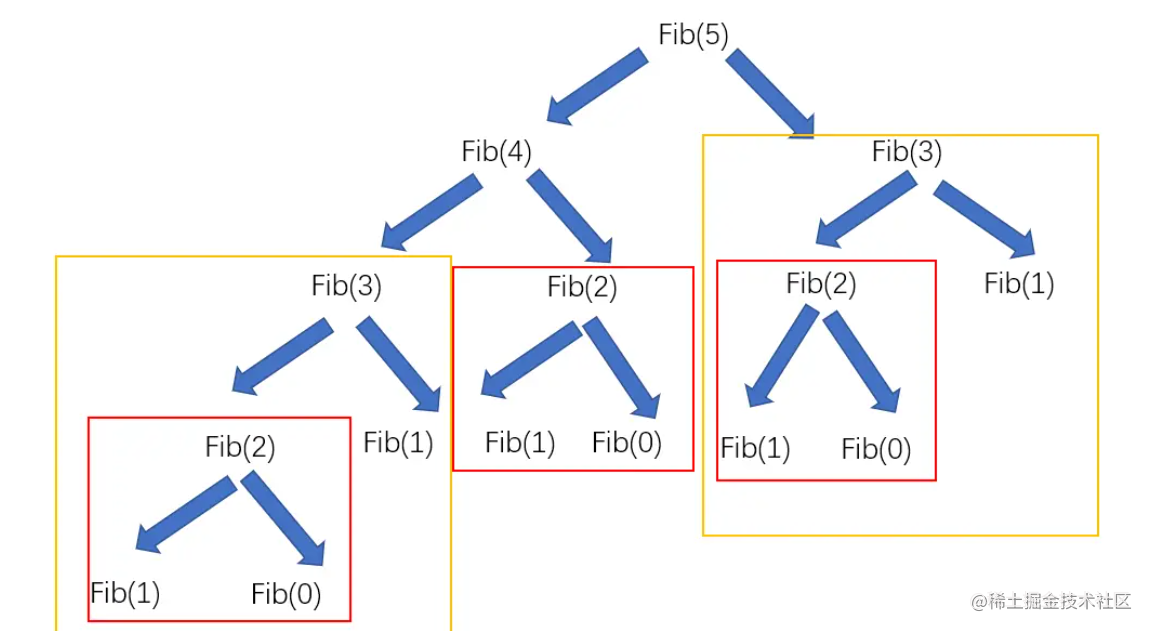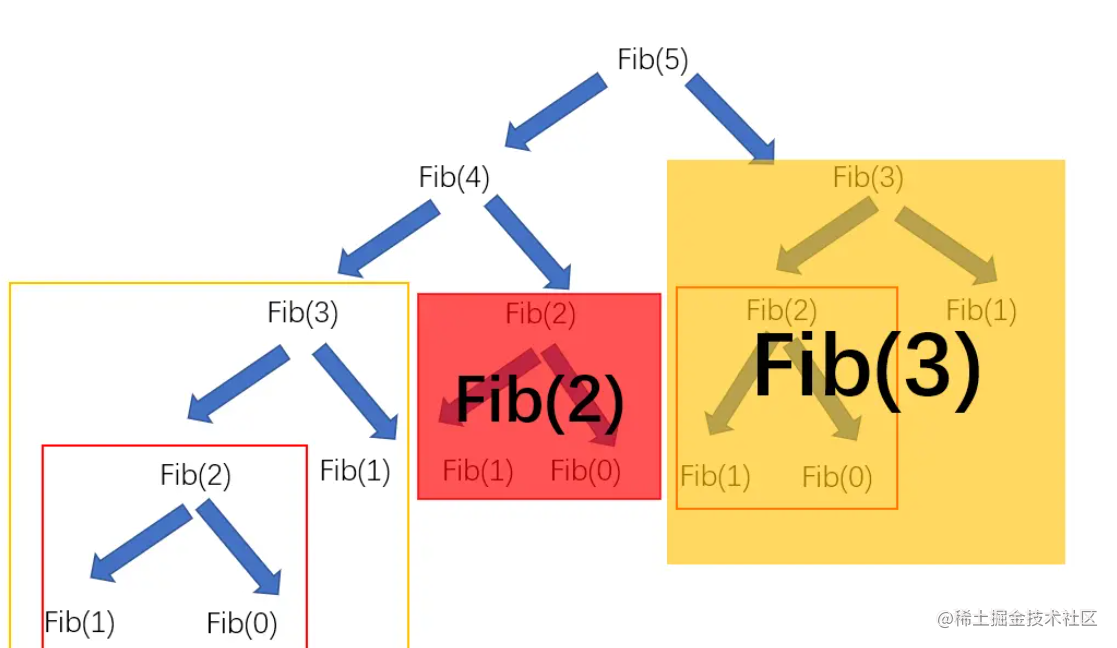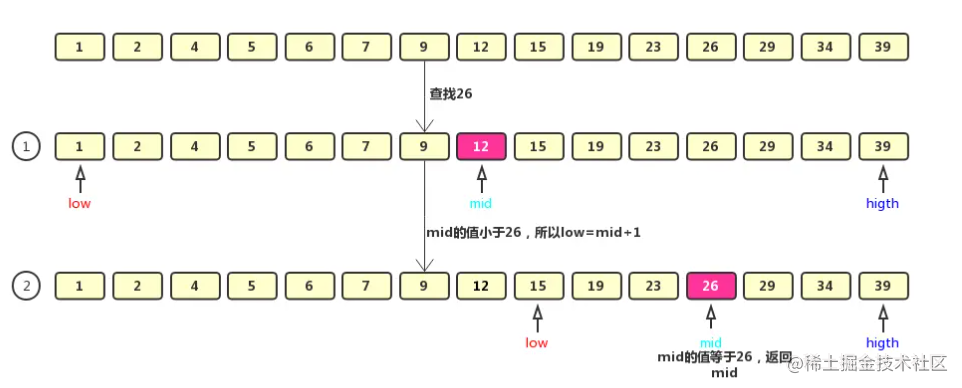# 引子

• 第一个月：一小兔1
• 第二个月：小兔1长成大兔1
• 第三个月：大兔1生了一只小兔2
• 第四个月：大兔1又生了一直小兔3，小兔2长成了大兔2
• ......# 斐波那契兔子数列问题建模

1，1，2，3，5，8，13，21，34，55，89

# 解析

## 法一：暴力递归

``````function fib(n) {
if( n === 0) return 0
if(n === 1) return 1
return fib(n-1) + fib(n-2)
};

let result = fib(12)
console.log(result) // 144## 递归 + 缓存``````//首先创建一个对象用来保存已经计算出的月数的兔子
let obj = {};
const fun = (e) => {
//如果这个月的兔子已经计算过了，那么直接返回，不再进行下一级的递归调用
if (obj[n]) {
return obj[n];
} else {
//如果找到第0个月或者第一个月，那么直接返回一只兔
if (e == 0 || e == 1) {
return 1;
}
//将递归求的每个月的兔子数量都保存下来
obj[n] = fun(e - 1) + fun(e - 2);
//返回当月的兔子
return obj[n];
}
};

## 法二：循环三指针

``````//第一个指针
let a = 0;
//第二个指针
let b = 0;
//第三个指针
let c = 0;
//月数
let n = 11;
for (let i = 0; i < n; i++) {
if (i === 0) {
a = 1;
b = 0;
} else if (i === 1) {
a = 1;
b = 1;
} else {
a = b;
b = c;
}
c = a + b;
}
console.log(c);

# 二分查找原理# 代码实现

``````//二分查找算法
let arr = [1, 2, 3, 4, 5, 6, 7, 8, 9, 10, 11, 12, 13];
//创造一个方法，传入需要查找的数组，开始查找的下标，结束查找的下标，查找的内容
const find = (arr, start, end, name) => {
//当下面的算法算出的开始下标大于结束下标的时候，证明查找不存在，直接返回-1
if (start > end) return -1;
//将查找的数组一分为二，求出中间数的位置
let mid = Math.floor((start + end) / 2);
if (arr[mid] === name) {
//如果这个中间数与查找的数相等，那么直接将这个位置返回
return mid;
} else if (arr[mid] > name) {
//如果这个中间数比查找的数大，那么说明这个数在中间数的左侧，就将左边的数组递归调用到这个函数里面，将结束下标改为中间下标减一
end = mid - 1;
return find(arr, start, end, name);
} else {
//如果这个中间数比查找的数小，那么说明这个数在中间数的右侧，就将右边的数组递归调用到这个函数里面，将结束下标改为中间下标加一
start = mid + 1;
return find(arr, start, end, name);
}
};
console.log(find(arr, 0, arr.length, 10));

# 递归

## 递归的概念

1. 直接调用自己
2. 间接调用自己 跳出结构,有了跳出才有结果

## 递归的思想

1. 如果有个函数foo,如果他是递归函数,到最后问题还是转换为函数foo的形式
2. 递归的思想就是将一个未知问题转换为一个已解决的问题来实现

## 递归的步骤(技巧)

1. 假设递归函数已经写好
2. 寻找递推关系
3. 将递推关系的结构转换为递归体
4. 将临界条件加入到递归体中